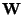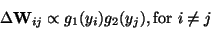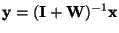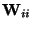Next: Non-linear decorrelation algorithms Up: Algorithms for ICA Previous: Preprocessing of the data

## Jutten-Hérault algorithm

The pioneering work in  was inspired by neural networks. Their algorithm was based on canceling the non-linear cross-correlations, see Section 4.3.3. The non-diagonal terms of the matrixare updated according to(33)

where g1 and g2 are some odd non-linear functions, and the yiare computed at every iteration as. The diagonal termsare set to zero. The yi then give, after convergence, estimates of the independent components. Unfortunately, the algorithm converges only under rather severe restrictions (see ).

Aapo Hyvarinen
1999-04-23